# Dynamic load of the carrying structure of an articulated wagon with new draft gear concepts

## Oleksij Fomin1, Alyona Lovska2, Václav Píštěk3, Pavel Kučera4

1Department of Cars and Carriage Facilities, State University of Infrastructure and Technologies, Kyiv, Ukraine

2Department of Wagons, Ukrainian State University оf Railway Transport, Kharkiv, Ukraine

3, 4Institute of Automotive Engineering, Brno University of Technology, Brno, Czech Republic

1Corresponding author

Vibroengineering PROCEDIA, Vol. 33, 2020, p. 84-89. https://doi.org/10.21595/vp.2020.21591
Received 10 July 2020; accepted 31 July 2020; published 19 October 2020

47th International Conference on Vibroengineering in Timisoara, Romania, October 19-20, 2020

Copyright © 2020 Oleksij Fomin, et al. This is an open access article distributed under the Creative Commons Attribution License, which permits unrestricted use, distribution, and reproduction in any medium, provided the original work is properly cited.
Views 75
CrossRef Citations 0
Abstract.

The article deals with a new draft gear concept proposed by the authors to reduce the dynamic load of wagons in operational modes. The peculiarity of the concept is transformation of the kinetic impact energy into the work of viscous resistance forces when the viscous fluid passes through the throttle openings of the piston. The concept can be introduced for the wagons, in which centre sills of the frames are of closed sections. The mathematic modelling was conducted for the dynamic load of articulated wagons with consideration of the draft gear concept. It was established that the use of the draft gear concept can lower the dynamic load of wagons by about 10 %. The research will encourage engineers to design modern structures of rolling stock units and increase their operational efficiency.

Keywords: articulated wagon, carrying structure, dynamics, strength, transport mechanics.

#### 1. Introduction

The competitive environment in the transportation market requires introduction of innovative engineering solutions to keep the leading position for the railway industry. Higher operational efficiency of the railway transport can be achieved by introduction of articulated wagons. The special feature of such wagons is the two-section carrying structure rested on three bogies. Such wagons have a number of considerable advantages in comparison with one-section ones; among them are lower production costs, lower vertical load on the frames, fewer number of required rolling stock units, etc.

Introduction of new innovative solutions in terms of the structure is essential for designing modern articulated wagons. It allows engineers to decrease the material capacity of wagons’ carrying structures, and, respectively, the production costs.

Possible improvements of the technical and economic characteristics for railway vehicles are considered in . But the study does not consider possible measures to decrease the dynamic load of wagons.

The structural analysis of the innovative rolling stock manufactured at UralVagonZavod is made in . The study considers modernization of rolling stock units and their components for higher operational efficiency. The measures for decreasing the dynamic load of the carrying structure of wagons under shunting impacts are not considered.

The structural analysis of BCNHL freight wagons is given in . The study outlines possible ways to improve the technical and economic parameters of wagons for higher operational efficiency.

The results of the structural and element analysis of a freight wagon are presented in . The calculation was made with the finite element method. The research is conducted by an example of a BOXN25 open wagon used by Indian Railways. Determination of the dynamic load of wagons and techniques for its decrease under shunting impacts are not considered in the studies.

The dynamic load of articulated wagons is determined in [5, 6]. The special feature of such wagons is circular-pipe structural elements. The values of dynamic load, obtained through mathematic modelling, are considered in the strength calculation for the carrying structures of wagons, but the measures to decrease the dynamic load of the carrying structure of wagons are not considered.

Prospects of implementation of new-generation materials in the railway wagon production are presented in . The study recounts some advantages of magnesium alloys used in the carrying systems of wagons.

However, the article does not cover peculiarities of the dynamic load of wagons produced of such materials under operational modes.

The structural peculiarities of a long-base flat wagon are presented in . The wagon has no frame-long centre sill, which is a special feature. The results of the strength calculation for the carrying structure of a flat wagon presented were realized in ANSYS software.

It should be mentioned that the design of the carrying structure of wagons considers the normative load values. Thus, the authors do not set the task to determine the dynamic load of the carrying structure of the wagon.

The objective of the study is determination of dynamic loads on the carrying structure of a circular-pipe wagon with consideration of the new draft gear concept instead of the automatic coupler CA-3. To achieve the objective, the following tasks were set:

- to propose techniques for lower dy11namic load on articulated wagons in operation;

- to conduct the mathematic modelling of dynamic loads on the carrying structure of an articulated wagon of circular pipes.

#### 2. New draft gear concept for decreasing the dynamic load

The authors proposed a draft gear concept for decreasing the dynamic load of wagons under operational modes (Fig. 1).

Fig. 1. The draft gear concept of an automatic coupler a) the concept’s components b) location on the wagon frame 1 – automatic couplers frame, 2 – wedge, 3 – adapter, 4 – circular-pipe centre sill, 5 – bottom, 6 – spring, 7 – telescopic element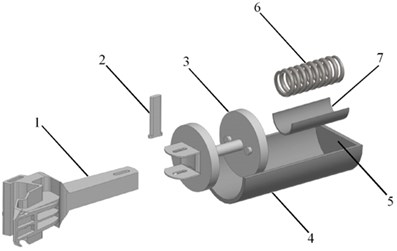a)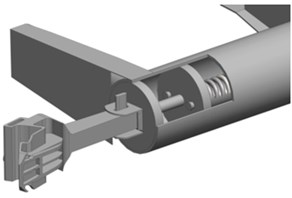b)

The kinetic impact energy is transformed into the work of viscous energy forces. The resistance appears when the piston transfers the viscous liquid through the throttle openings by the operational principle of a hydraulic damper. The system returns to its initial state with the release spring in the telescopic element.

It should be mentioned, that such a draft gear concept can be used for railway vehicles with closed-section centre sills. This engineering solution can be used, e.g. for wagons with carrying elements of circular pipes (see Fig. 2).

The dynamic load of the carrying structure of the wagon with the draft gear concept was determined due to a mathematic functional notation of the process of dynamic load under the longitudinal force on the front draft gear stop (tension – jerk). The calculation was conducted by an example of an articulated flat wagon as one of the most popular types of articulated wagons in operation.

Fig. 2. The spatial computer models of freight wagons: a) flat wagon, b) open wagon, c) boxcar, d) hopper wagon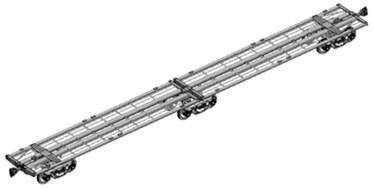a)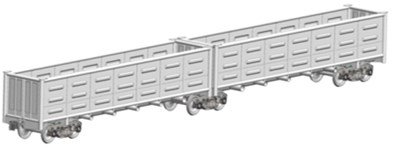b)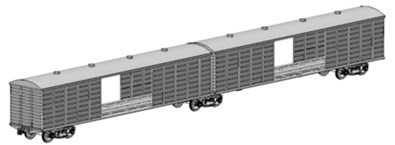c)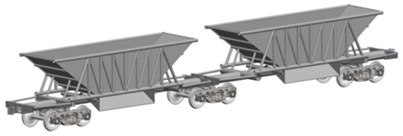d)

The design diagram is presented in Fig. 3.

Fig. 3. The design diagram of an articulated flat wagon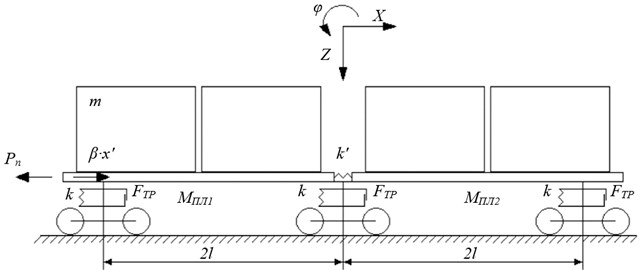(1)
${M}_{w}^{\text{'}}\bullet {\stackrel{¨}{x}}_{{w}_{1}}+{M}_{{W}_{1}}\bullet h\bullet {\stackrel{¨}{\phi }}_{{W}_{1}}+{k}^{\text{'}}\left({x}_{{w}_{1}}-{x}_{{w}_{2}}\right)={P}_{n}-\beta \bullet {\stackrel{˙}{x}}_{{w}_{1}},$
(2)
${I}_{{w}_{1}}\bullet {\stackrel{¨}{\phi }}_{{w}_{1}}+{M}_{{W}_{1}}\bullet h\bullet {\stackrel{¨}{x}}_{{w}_{1}}-g\bullet {\phi }_{{w}_{1}}\bullet {M}_{{W}_{1}}\bullet h=l\bullet {F}_{FR}\left(sign{\stackrel{˙}{∆}}_{1}^{{W}_{1}}-sign{\stackrel{˙}{∆}}_{2}^{{W}_{1}}\right)+l\left({k}_{1}\bullet {\stackrel{˙}{∆}}_{1}^{{W}_{1}}-{k}_{2}\bullet {\stackrel{˙}{∆}}_{2}^{{W}_{1}}\right),$
(3)
${M}_{{w}_{1}}\bullet {\stackrel{¨}{z}}_{{w}_{1}}={k}_{1}\bullet {∆}_{1}^{{W}_{1}}+{k}_{2}\bullet {∆}_{2}^{{W}_{1}}-{F}_{FR}\left(sign{\stackrel{˙}{∆}}_{1}^{{W}_{1}}-sign{\stackrel{˙}{∆}}_{2}^{{W}_{1}}\right),$
(4)
${m}_{i}\bullet {\stackrel{¨}{x}}_{{w}_{1}}+\left({m}_{i}\bullet {z}_{{c}_{i}}\right)\bullet {\stackrel{¨}{\phi }}_{{w}_{1}}=0,$
(5)
${I}_{i}\bullet {\stackrel{¨}{\phi }}_{{w}_{1}}+\left({m}_{i}\bullet {z}_{{c}_{i}}\right)\bullet {\stackrel{¨}{x}}_{{w}_{1}}-g\left({m}_{i}\bullet {z}_{{c}_{i}}\right)\bullet {\phi }_{{w}_{1}}=0,$
(6)
${m}_{i}\bullet {\stackrel{¨}{z}}_{{w}_{1}}=0,$
(7)
${M}_{{w}_{2}}^{\text{'}}\bullet {\stackrel{¨}{x}}_{{w}_{2}}+{M}_{{W}_{2}}\bullet h\bullet {\stackrel{¨}{\phi }}_{{W}_{2}}-{k}^{\text{'}}\left({x}_{{w}_{1}}-{x}_{{w}_{2}}\right)=0,$
(8)
${I}_{{w}_{2}}\bullet {\stackrel{¨}{\phi }}_{{w}_{2}}+{M}_{{W}_{2}}\bullet h\bullet {\stackrel{¨}{x}}_{{w}_{2}}-g\bullet {\phi }_{{w}_{2}}\bullet {M}_{{W}_{2}}\bullet h=l\bullet {F}_{FR}\left(sign{\stackrel{˙}{∆}}_{1}^{{W}_{2}}-sign{\stackrel{˙}{∆}}_{2}^{{W}_{2}}\right)+l\left({k}_{1}\bullet {\stackrel{˙}{∆}}_{1}^{{W}_{2}}-{k}_{2}\bullet {\stackrel{˙}{∆}}_{2}^{{W}_{2}}\right),$
(9)
${M}_{{w}_{2}}\bullet {\stackrel{¨}{z}}_{{w}_{22}}={k}_{1}\bullet {∆}_{1}^{{W}_{2}}+{k}_{2}\bullet {∆}_{2}^{{W}_{2}}-{F}_{FR}\left(sign{\stackrel{˙}{∆}}_{1}^{{W}_{2}}-sign{\stackrel{˙}{∆}}_{2}^{{W}_{2}}\right),$
(10)
${m}_{i}\bullet {\stackrel{¨}{x}}_{{w}_{2}}+\left({m}_{i}\bullet {z}_{{c}_{i}}\right)\bullet {\stackrel{¨}{\phi }}_{{w}_{2}}=0,$
(11)
${I}_{i}\bullet {\stackrel{¨}{\phi }}_{{w}_{2}}+\left({m}_{i}\bullet {z}_{{c}_{i}}\right)\bullet {\stackrel{¨}{x}}_{{w}_{2}}-g\left({m}_{i}\bullet {z}_{{c}_{i}}\right)\bullet {\phi }_{{w}_{2}}=0,$
(12)
${m}_{i}\bullet {\stackrel{¨}{z}}_{{w}_{2}}=0,$

where ${∆}_{1}^{i}={z}_{{w}_{1}}-l\bullet {\phi }_{{w}_{1}}$, ${∆}_{2}^{i}={z}_{{w}_{2}}+l\bullet {\phi }_{{w}_{2}}$, ${M}_{Wi}^{\text{'}}$– gross mass of the $i$-th section of a flat wagon; ${M}_{Wi}$ – mass of the carrying structure of the $i$-th section of a flat wagon; ${I}_{Wi}$ – inertia moment of the $i$-th section of a flat wagon; ${P}_{n}$ – longitudinal force on the automatic coupler; $\beta$ – viscous resistance coefficient formed by the draft gear concept; $l$ – half-base of a flat-wagon section; ${F}_{FR}$ – absolute value of the dry force in a spring group; ${k}^{\text{'}}$ – rigidity of connection between the sections; ${k}_{1}$, ${k}_{2}$ – rigidities of the springs in the spring group of a flat wagon bogie (of Model 18-100); ${m}_{i}$ – container mass; ${z}_{ci}$ – height of the container’s gravity centre; ${I}_{i}$ – inertia moment of the $i$-th container; ${x}_{i}$, ${\phi }_{i}$, ${z}_{i}$ – coordinates corresponding to displacements of the flat wagon sections relative to the appropriate axles.

The research was conducted in the plane coordinates. The sections were elastically interacted. The longitudinal load on the carrying structure of a flat wagon was taken equal to 2.5 МN [9, 10]. The initial displacements and speeds were taken equal to zero [11-16]. Differential Eq. (1-12) were solved in MathCad environment by the Runge-Kutta method [17-23].

The results of the calculation showed than the accelerations on the carrying structure of the first section of a flat wagon from the force action, were 34.9 m/s², and on the second section – 34.2 m/s² (Fig. 4). The viscous resistance coefficient formed by the draft gear concept should exceed 70 kN/s∙m.

Fig. 4. The accelerations on the carrying structure of an articulated flat wagon a) the first section of a flat wagon from the longitudinal force, b) the second section of a flat wagon from the longitudinal force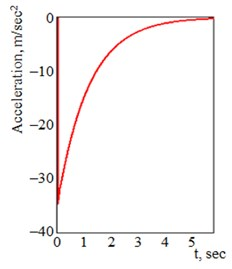a)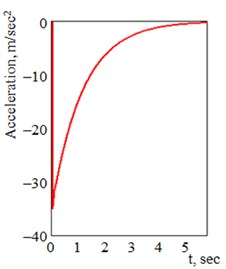b)

Thus, the use of the draft gear concept for an articulated flat wagon makes it possible to reduce the dynamic load by about 10 %.

The calculations were also made for other types of articulated wagons of circular pipes. The numerical values of accelerations are given in Table 1.

Table 1. The numerical values of accelerations on the carrying structure of an articulated wagon of circular pipes

 Wagon type Accelerations on the first section from the force action Accelerations on the second section from the force action Open wagon 28.3 27.9 Boxcar 25.7 25.2 Hopper wagon 28.5 28.1

The research conducted proved the efficiency of the draft gear concept for railway vehicles.

#### 3. Conclusions

The authors proposed a draft gear concept for lower dynamic load of wagons under operational modes. This concept can be used for wagons with closed-section centre sills.

The study deals with the mathematical modelling of the dynamic load on the carrying structure of an articulated flat wagon of circular pipes. The results of the calculation showed that the accelerations on the carrying structure the first section of a flat wagon from the action force were 34.9 m/s2, and on the second section – 34.2 m/s2.

It was established that the use of the draft gear concept for articulated wagons can help decrease the dynamic load by about 10 %.

The research will encourage engineers to design modern structures for railway vehicles of better operational efficiency.

#### Acknowledgements

The present study was conducted within the framework of the scientific topic of young scientists “Innovative Principles of Creation of Resource-Saving Structures of Railroad Cars by Taking into Account the Refined Dynamic Loads and Functional-Adaptive Flash Concepts”, which is funded by the state budget of Ukraine in 2020 and the authors gratefully acknowledge funding from the Specific research on BUT FSI-S-20-6267.

1. Boronenko Yu P. The strategic objectives of wagon design in the development of heavy traffic. Transport of the Russian Federation, Vol. 5, Issue 48, 2013, p. 68-73, (in Russian). [Search CrossRef]
2. Innovative rolling stock manufactured by „Uralvagonzavod” for railways with a space of 1520 mm. Transport of the Russian Federation, Vol. 3, Issue 28, 2010, p. 20-21, (in Russian). [Search CrossRef]
3. Chandra Prakash Shukla, Bharti P. K. Study and analysis of doors of BCNHL wagons. International Journal of Engineering Research and Technology (IJERT), Vol. 4, Issue 4, 2015, p. 1195-1200. [Publisher]
4. Harak S. S., Sharma S. C., Harsha S. P. Structural dynamic analysis of freight railway wagon using finite element method. Procedia Materials Science, Vol. 6, 2014, p. 1891-1898. [Publisher]
5. Fomin O., Lovska A., Píštěk V., Kučera P. Dynamic load computational modelling of containers placed on a flat wagon at railroad ferry transportation. Vibroengineering Procedia, Vol. 29, 2019, p. 118-123. [Publisher]
6. Fomin O., Lovska A., Píštěk V., Kučera P. Research of stability of containers in the combined trains during transportation by railroad ferry. MM Science Journal, 2020, p. 3728-3733. [Publisher]
7. Woo G. L., Jungseok K., Sun S. J., Jae Y. L. The next generation material for lightweight railway car body structures: Magnesium alloys. Proceeding of the institution of Mechanical Engineers Part F. Journal of Rail and Rapid Transit, Vol. 232, Issue 1, 2016, p. 25-42. [Publisher]
8. Šťastniak P., Kurčík P., Pavlík A. Design of a new railway wagon for intermodal transport with the adaptable loading platform. MATEC Web of Conferences, 2018. [Publisher]
9. Freight Wagons. General Requirements for the Calculations and Design of New and Upgraded 1520 mm (non-self-propelled) Railcars. DSTU 7598: 2014, 2014, (in Ukrainian). [Search CrossRef]
10. Freight Wagons. Strength and Dynamic Quality Requirements. GOST 33211-2014, 2014, (in Russian). [Search CrossRef]
11. Aleksandrowicz, P. Verifying a truck collision applying the SDC method. Proceedings of 58th International Conference of Machine Design Departments, Praque, Czech Republic, 2017, p. 14-19. [Search CrossRef]
12. Kostek R., Aleksandrowicz P. Effect of contact parameters on the pattern of vehicle collisions with a round pillar. Proceedings of 23rd International Conference Engineering Mechanics, Svratka, Czech Republic, 2017, p. 490-493. [Search CrossRef]
13. Kostek R., Aleksandrowicz P. Simulation of the right-angle car collision based on identified parameters. IOP Conference Series, 2017. [Publisher]
14. Sapronova S., Tkachenko V., Fomin O., Hatchenko V., Maliuk S. Research on the safety factor against derailment of railway vehicles. Eastern-European Journal of Enterprise Technologies, Vol. 6, Issues 7(90), 2017, p. 19-25. [Publisher]
15. Okorokov A. M., Fomin O. V., Lovska A. O., Vernigora R. V., Zhuravel I. L., Fomin V. V. Research into a possibility to prolong the time of operation of universal semi-wagon bodies that have exhausted their standard resource. Eastern-European Journal of Enterprise Technologies, Vol. 3 Issues 7(93), 2018, p. 20-26. [Publisher]
16. Lovskaya A., Rybin А. The study of dynamic load on a wagon-platform at a shunting collision. Eastern-European Journal of Enterprise Technologies, Vol. 3, 2016, p. 4-8. [Publisher]
17. Vatulia G., Falendysh A., Orel Y., Pavliuchenkov M. Structural Improvements in a Tank Wagon with modern software packages. Procedia Engineering, Vol. 187, 2017, p. 301-307. [Publisher]
18. Kitov Y., Verevicheva M., Vatulia G., Orel Y., Deryzemlia S. Design solutions for structures with optimal internal stress distribution. MATEC Web of Conferences, Vol. 133, Issues 1-3, 2017, p. 0300. [Publisher]
19. Novotný P., Drápal L., Prokop A. Řehák, K. Modelling the powertrain rubber coupling under dynamic conditions. Journal of Vibroengineering, Vol. 19, Issue 5, 2017, p. 3710-3719. [Publisher]
20. Novotný P., Dlugoš J., Prokop A. Řehák, K., Raffai P. Effective computational model for a solution of turbocharger rotor dynamics. Journal of Vibroengineering, Vol. 19, Issue 2, 2017, p. 724-736. [Publisher]
21. Drápal L., Novotný P. Torsional vibration analysis of crank train with low friction losses. Journal of Vibroengineering, Vol. 19, Issue 8, 2017, p. 5691-5701. [Publisher]
22. Kučera P., Píštěk V. Truck vibrations caused by rotating shaft deflection. Journal of Vibroengineering, Vol. 19, Issue 7, 2017, p. 5361-5373. [Publisher]
23. Píštěk V., Klimeš L., Mauder T., Kučera P. Optimal design of structure in rheological models: an automotive application to dampers with high viscosity silicone fluids. Journal of Vibroengineering, Vol. 19, Issue 6, 2017, p. 4459-4470. [Publisher]